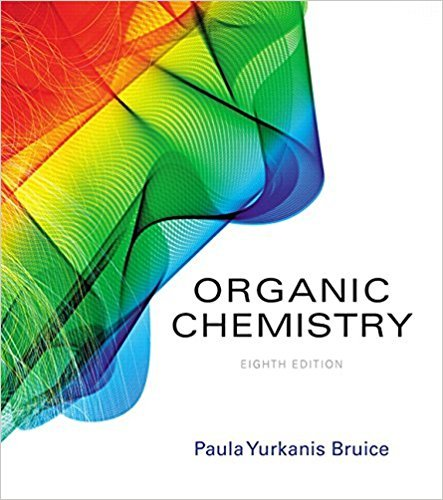×
Get Full Access to Organic Chemistry - 8 Edition - Chapter 8 - Problem 106
Get Full Access to Organic Chemistry - 8 Edition - Chapter 8 - Problem 106

×

# Solved: While attempting to recrystallize maleic anhydride, a student dissolved it inISBN: 9780134042282 482

## Solution for problem 106 Chapter 8

Organic Chemistry | 8th Edition

• Textbook Solutions
• 2901 Step-by-step solutions solved by professors and subject experts
• Get 24/7 help from StudySoup virtual teaching assistantsOrganic Chemistry | 8th Edition

4 5 1 305 Reviews
24
3
Problem 106

While attempting to recrystallize maleic anhydride, a student dissolved it in freshly distilled cyclopentadiene rather than in freshly distilled cyclopentane.Was her recrystallization successful?

Step-by-Step Solution:
Step 1 of 3

Chemistry of Solutions Lecture 11 Tuesday, October 4, 2016 Exercise Continued: calculate the pH of a 1.00x10 M H SO solution, for2the 4econd dissociation of H SO2. 4 The major species are H + , HSO - , and H O . (aq) 4 (aq) 2 (l) -2 -3 K a 1.2 x 10 , don't approximate because K must be at aeast 10 smaller than the initial concentration of H SO 2 4 1.2x10 = [HSO ] [H ]4/ [H SO ] 2 4 -2 -2 -2 1.2x10 = (1.0x10 + x) (x) / (1.0x10 – x) (1.2x10 )(1.0x10 – x) = (1.0x10 + x ) -2 2 Using the Quadratic Equation: a = 1, b = 2.2x10 , c = -1.2x10 . -4 -2 -

Step 2 of 3

Step 3 of 3

##### ISBN: 9780134042282

This full solution covers the following key subjects: . This expansive textbook survival guide covers 127 chapters, and 2116 solutions. This textbook survival guide was created for the textbook: Organic Chemistry, edition: 8. Since the solution to 106 from 8 chapter was answered, more than 224 students have viewed the full step-by-step answer. The full step-by-step solution to problem: 106 from chapter: 8 was answered by , our top Chemistry solution expert on 03/16/18, 04:59PM. The answer to “While attempting to recrystallize maleic anhydride, a student dissolved it in freshly distilled cyclopentadiene rather than in freshly distilled cyclopentane.Was her recrystallization successful?” is broken down into a number of easy to follow steps, and 23 words. Organic Chemistry was written by and is associated to the ISBN: 9780134042282.

Unlock Textbook Solution